GFG App
Open AppBrowser
Continue

# Breadth First Search or BFS for a Graph

The breadth-first search (BFS) algorithm is used to search a tree or graph data structure for a node that meets a set of criteria. It starts at the tree’s root or graph and searches/visits all nodes at the current depth level before moving on to the nodes at the next depth level. Breadth-first search can be used to solve many problems in graph theory.

## Relation between BFS for Graph and Tree traversal:

Breadth-First Traversal (or Search) for a graph is similar to the Breadth-First Traversal of a tree (See method 2 of this post).

The only catch here is, that, unlike trees, graphs may contain cycles, so we may come to the same node again. To avoid processing a node more than once, we divide the vertices into two categories:

• Visited and
• Not visited.

A boolean visited array is used to mark the visited vertices. For simplicity, it is assumed that all vertices are reachable from the starting vertex. BFS uses a queue data structure for traversal.

• Step 1: Consider the graph you want to navigate.
• Step 2: Select any vertex in your graph (say v1), from which you want to traverse the graph.
• Step 3: Utilize the following two data structures for traversing the graph.
• Visited array(size of the graph)
• Queue data structure
• Step 4: Add the starting vertex to the visited array, and afterward, you add v1’s adjacent vertices to the queue data structure.
• Step 5: Now using the FIFO concept, remove the first element from the queue, put it into the visited array, and then add the adjacent vertices of the removed element to the queue.
• Step 6: Repeat step 5 until the queue is not empty and no vertex is left to be visited.

Examples:

In the following graph, we start traversal from vertex 2.When we come to vertex 0, we look for all adjacent vertices of it.

• 2 is also an adjacent vertex of 0.
• If we don’t mark visited vertices, then 2 will be processed again and it will become a non-terminating process.

There can be multiple BFS traversals for a graph. Different BFS traversals for the above graph :
2, 3, 0, 1
2, 0, 3, 1

Recommended Practice

## Implementation of BFS traversal on Graph:

### Pseudocode for BFS:

// Here, Graph is the graph that we already have and X is the source node
Let Q be the queue
Q.enqueue( X )     // Inserting source node X into the queue
Mark X node as visited.

While ( Q is not empty )
Y = Q.dequeue( )     // Removing the front node from the queue

Process all the neighbors of Y, For all the neighbors Z of Y
If Z is not visited:
Q. enqueue( Z )     // Stores Z in Q
Mark Z as visited

Follow the below method to implement BFS traversal.

• Declare a queue and insert the starting vertex.
• Initialize a visited array and mark the starting vertex as visited.
• Follow the below process till the queue becomes empty:
• Remove the first vertex of the queue.
• Mark that vertex as visited.
• Insert all the unvisited neighbors of the vertex into the queue.

Illustration:

Step1: Initially queue and visited arrays are empty.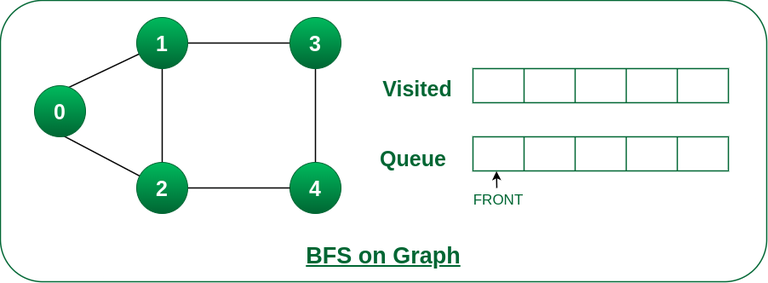Queue and visited arrays are empty initially.

Step2: Push node 0 into queue and mark it visited.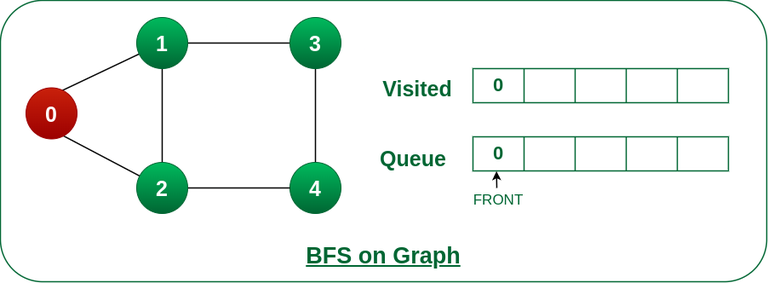Push node 0 into queue and mark it visited.

Step 3: Remove node 0 from the front of queue and visit the unvisited neighbours and push them into queue.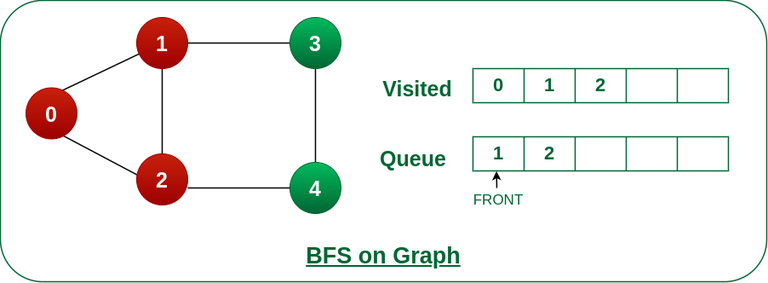Remove node 0 from the front of queue and visited the unvisited neighbours and push into queue.

Step 4: Remove node 1 from the front of queue and visit the unvisited neighbours and push them into queue.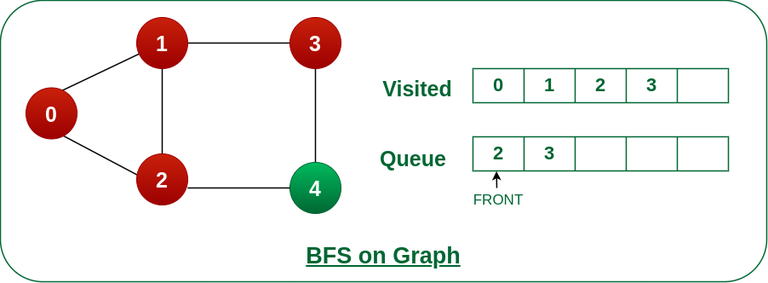Remove node 1 from the front of queue and visited the unvisited neighbours and push

Step 5: Remove node 2 from the front of queue and visit the unvisited neighbours and push them into queue.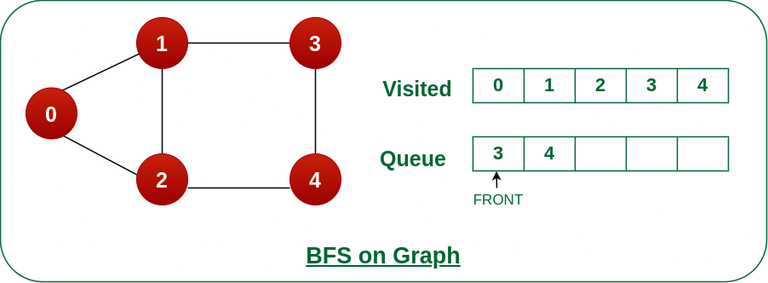Remove node 2 from the front of queue and visit the unvisited neighbours and push them into queue.

Step 6: Remove node 3 from the front of queue and visit the unvisited neighbours and push them into queue.
As we can see that every neighbours of node 3 is visited, so move to the next node that are in the front of the queue.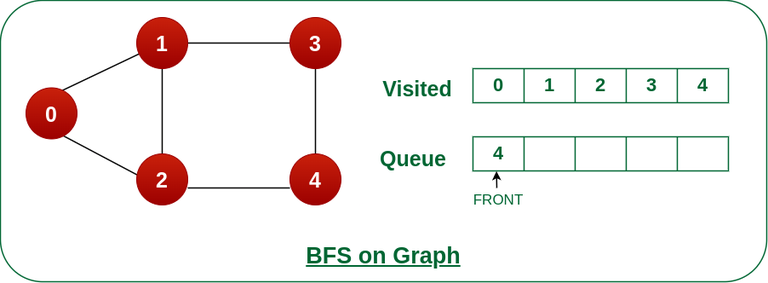Remove node 3 from the front of queue and visit the unvisited neighbours and push them into queue.

Steps 7: Remove node 4 from the front of queue and visit the unvisited neighbours and push them into queue.
As we can see that every neighbours of node 4 are visited, so move to the next node that is in the front of the queue.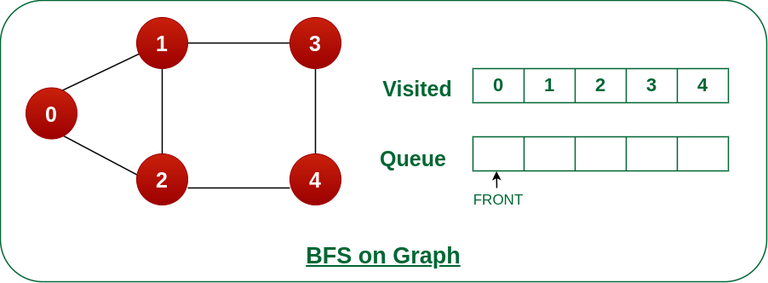Remove node 4 from the front of queue and visit the unvisited neighbours and push them into queue.

Now, Queue becomes empty, So, terminate these process of iteration.

The implementation uses an adjacency list representation of graphs. STL‘s list container stores lists of adjacent nodes and the queue of nodes needed for BFS traversal.

## C

 `#include ` `#include ` `#include `   `#define MAX_VERTICES 50`   `// This struct represents a directed graph using` `// adjacency list representation` `typedef` `struct` `Graph_t {` `    `  `    ``// No. of vertices` `    ``int` `V;` `    ``bool` `adj[MAX_VERTICES][MAX_VERTICES];` `} Graph;`   `// Constructor` `Graph* Graph_create(``int` `V)` `{` `    ``Graph* g = ``malloc``(``sizeof``(Graph));` `    ``g->V = V;`   `    ``for` `(``int` `i = 0; i < V; i++) {` `        ``for` `(``int` `j = 0; j < V; j++) {` `            ``g->adj[i][j] = ``false``;` `        ``}` `    ``}`   `    ``return` `g;` `}`   `// Destructor` `void` `Graph_destroy(Graph* g) { ` `    ``free``(g);` `}`   `// function to add an edge to graph` `void` `Graph_addEdge(Graph* g, ``int` `v, ``int` `w)` `{` `    ``// Add w to v’s list.` `    ``g->adj[v][w] = ``true``;` `}`   `// Prints BFS traversal from a given source s` `void` `Graph_BFS(Graph* g, ``int` `s)` `{` `    ``// Mark all the vertices as not visited` `    ``bool` `visited[MAX_VERTICES];` `    ``for` `(``int` `i = 0; i < g->V; i++) {` `        ``visited[i] = ``false``;` `    ``}`   `    ``// Create a queue for BFS` `    ``int` `queue[MAX_VERTICES];` `    ``int` `front = 0, rear = 0;`   `    ``// Mark the current node as visited and enqueue it` `    ``visited[s] = ``true``;` `    ``queue[rear++] = s;`   `    ``while` `(front != rear) {` `        ``// Dequeue a vertex from queue and print it` `        ``s = queue[front++];` `        ``printf``(``"%d "``, s);`   `        ``// Get all adjacent vertices of the dequeued` `        ``// vertex s. If a adjacent has not been visited,` `        ``// then mark it visited and enqueue it` `        ``for` `(``int` `adjacent = 0; adjacent < g->V;` `             ``adjacent++) {` `            ``if` `(g->adj[s][adjacent] && !visited[adjacent]) {` `                ``visited[adjacent] = ``true``;` `                ``queue[rear++] = adjacent;` `            ``}` `        ``}` `    ``}` `}`   `// Driver code` `int` `main()` `{` `    ``// Create a graph` `    ``Graph* g = Graph_create(4);` `    ``Graph_addEdge(g, 0, 1);` `    ``Graph_addEdge(g, 0, 2);` `    ``Graph_addEdge(g, 1, 2);` `    ``Graph_addEdge(g, 2, 0);` `    ``Graph_addEdge(g, 2, 3);` `    ``Graph_addEdge(g, 3, 3);`   `    ``printf``(``"Following is Breadth First Traversal "` `           ``"(starting from vertex 2) \n"``);` `    ``Graph_BFS(g, 2);` `    ``Graph_destroy(g);`   `    ``return` `0;` `}`

## C++

 `// C++ code to print BFS traversal from a given` `// source vertex`   `#include ` `using` `namespace` `std;`   `// This class represents a directed graph using` `// adjacency list representation` `class` `Graph {`   `    ``// No. of vertices` `    ``int` `V;`   `    ``// Pointer to an array containing adjacency lists` `    ``vector > adj;`   `public``:` `    ``// Constructor` `    ``Graph(``int` `V);`   `    ``// Function to add an edge to graph` `    ``void` `addEdge(``int` `v, ``int` `w);`   `    ``// Prints BFS traversal from a given source s` `    ``void` `BFS(``int` `s);` `};`   `Graph::Graph(``int` `V)` `{` `    ``this``->V = V;` `    ``adj.resize(V);` `}`   `void` `Graph::addEdge(``int` `v, ``int` `w)` `{` `    ``// Add w to v’s list.` `    ``adj[v].push_back(w);` `}`   `void` `Graph::BFS(``int` `s)` `{` `    ``// Mark all the vertices as not visited` `    ``vector<``bool``> visited;` `    ``visited.resize(V, ``false``);`   `    ``// Create a queue for BFS` `    ``list<``int``> queue;`   `    ``// Mark the current node as visited and enqueue it` `    ``visited[s] = ``true``;` `    ``queue.push_back(s);`   `    ``while` `(!queue.empty()) {` `        `  `        ``// Dequeue a vertex from queue and print it` `        ``s = queue.front();` `        ``cout << s << ``" "``;` `        ``queue.pop_front();`   `        ``// Get all adjacent vertices of the dequeued` `        ``// vertex s. If a adjacent has not been visited,` `        ``// then mark it visited and enqueue it` `        ``for` `(``auto` `adjacent : adj[s]) {` `            ``if` `(!visited[adjacent]) {` `                ``visited[adjacent] = ``true``;` `                ``queue.push_back(adjacent);` `            ``}` `        ``}` `    ``}` `}`   `// Driver code` `int` `main()` `{` `    ``// Create a graph given in the above diagram` `    ``Graph g(4);` `    ``g.addEdge(0, 1);` `    ``g.addEdge(0, 2);` `    ``g.addEdge(1, 2);` `    ``g.addEdge(2, 0);` `    ``g.addEdge(2, 3);` `    ``g.addEdge(3, 3);`   `    ``cout << ``"Following is Breadth First Traversal "` `         ``<< ``"(starting from vertex 2) \n"``;` `    ``g.BFS(2);`   `    ``return` `0;` `}`

## Java

 `// Java program to print BFS traversal from a given source` `// vertex. BFS(int s) traverses vertices reachable from s.`   `import` `java.io.*;` `import` `java.util.*;`   `// This class represents a directed graph using adjacency` `// list representation` `class` `Graph {`   `    ``// No. of vertices` `    ``private` `int` `V;`   `    ``// Adjacency Lists` `    ``private` `LinkedList adj[];`   `    ``// Constructor` `    ``Graph(``int` `v)` `    ``{` `        ``V = v;` `        ``adj = ``new` `LinkedList[v];` `        ``for` `(``int` `i = ``0``; i < v; ++i)` `            ``adj[i] = ``new` `LinkedList();` `    ``}`   `    ``// Function to add an edge into the graph` `    ``void` `addEdge(``int` `v, ``int` `w) { adj[v].add(w); }`   `    ``// prints BFS traversal from a given source s` `    ``void` `BFS(``int` `s)` `    ``{` `        ``// Mark all the vertices as not visited(By default` `        ``// set as false)` `        ``boolean` `visited[] = ``new` `boolean``[V];`   `        ``// Create a queue for BFS` `        ``LinkedList queue` `            ``= ``new` `LinkedList();`   `        ``// Mark the current node as visited and enqueue it` `        ``visited[s] = ``true``;` `        ``queue.add(s);`   `        ``while` `(queue.size() != ``0``) {` `            `  `            ``// Dequeue a vertex from queue and print it` `            ``s = queue.poll();` `            ``System.out.print(s + ``" "``);`   `            ``// Get all adjacent vertices of the dequeued` `            ``// vertex s If a adjacent has not been visited,` `            ``// then mark it visited and enqueue it` `            ``Iterator i = adj[s].listIterator();` `            ``while` `(i.hasNext()) {` `                ``int` `n = i.next();` `                ``if` `(!visited[n]) {` `                    ``visited[n] = ``true``;` `                    ``queue.add(n);` `                ``}` `            ``}` `        ``}` `    ``}`   `    ``// Driver code` `    ``public` `static` `void` `main(String args[])` `    ``{` `        ``Graph g = ``new` `Graph(``4``);` `        ``g.addEdge(``0``, ``1``);` `        ``g.addEdge(``0``, ``2``);` `        ``g.addEdge(``1``, ``2``);` `        ``g.addEdge(``2``, ``0``);` `        ``g.addEdge(``2``, ``3``);` `        ``g.addEdge(``3``, ``3``);`   `        ``System.out.println(` `            ``"Following is Breadth First Traversal "` `            ``+ ``"(starting from vertex 2)"``);`   `        ``g.BFS(``2``);` `    ``}` `}`   `// This code is contributed by Aakash Hasija`

## Python3

 `# Python3 Program to print BFS traversal` `# from a given source vertex. BFS(int s)` `# traverses vertices reachable from s.`   `from` `collections ``import` `defaultdict`     `# This class represents a directed graph` `# using adjacency list representation` `class` `Graph:`   `    ``# Constructor` `    ``def` `__init__(``self``):`   `        ``# Default dictionary to store graph` `        ``self``.graph ``=` `defaultdict(``list``)`   `    ``# Function to add an edge to graph` `    ``def` `addEdge(``self``, u, v):` `        ``self``.graph[u].append(v)`   `    ``# Function to print a BFS of graph` `    ``def` `BFS(``self``, s):`   `        ``# Mark all the vertices as not visited` `        ``visited ``=` `[``False``] ``*` `(``max``(``self``.graph) ``+` `1``)`   `        ``# Create a queue for BFS` `        ``queue ``=` `[]`   `        ``# Mark the source node as` `        ``# visited and enqueue it` `        ``queue.append(s)` `        ``visited[s] ``=` `True`   `        ``while` `queue:`   `            ``# Dequeue a vertex from` `            ``# queue and print it` `            ``s ``=` `queue.pop(``0``)` `            ``print``(s, end``=``" "``)`   `            ``# Get all adjacent vertices of the` `            ``# dequeued vertex s. If a adjacent` `            ``# has not been visited, then mark it` `            ``# visited and enqueue it` `            ``for` `i ``in` `self``.graph[s]:` `                ``if` `visited[i] ``=``=` `False``:` `                    ``queue.append(i)` `                    ``visited[i] ``=` `True`     `# Driver code` `if` `__name__ ``=``=` `'__main__'``:`   `    ``# Create a graph given in` `    ``# the above diagram` `    ``g ``=` `Graph()` `    ``g.addEdge(``0``, ``1``)` `    ``g.addEdge(``0``, ``2``)` `    ``g.addEdge(``1``, ``2``)` `    ``g.addEdge(``2``, ``0``)` `    ``g.addEdge(``2``, ``3``)` `    ``g.addEdge(``3``, ``3``)`   `    ``print``(``"Following is Breadth First Traversal"` `          ``" (starting from vertex 2)"``)` `    ``g.BFS(``2``)`   `# This code is contributed by Neelam Yadav`

## C#

 `// C# program to print BFS traversal from a given source` `// vertex. BFS(int s) traverses vertices reachable from s.`   `using` `System;` `using` `System.Collections.Generic;` `using` `System.Linq;` `using` `System.Text;`   `// This class represents a directed graph ` `// using adjacency list representation` `class` `Graph {`   `    ``// No. of vertices` `    ``private` `int` `_V;`   `    ``// Adjacency Lists` `    ``LinkedList<``int``>[] _adj;`   `    ``public` `Graph(``int` `V)` `    ``{` `        ``_adj = ``new` `LinkedList<``int``>[ V ];` `        ``for` `(``int` `i = 0; i < _adj.Length; i++) {` `            ``_adj[i] = ``new` `LinkedList<``int``>();` `        ``}` `        ``_V = V;` `    ``}`   `    ``// Function to add an edge into the graph` `    ``public` `void` `AddEdge(``int` `v, ``int` `w)` `    ``{` `        ``_adj[v].AddLast(w);` `    ``}`   `    ``// Prints BFS traversal from a given source s` `    ``public` `void` `BFS(``int` `s)` `    ``{`   `        ``// Mark all the vertices as not` `        ``// visited(By default set as false)` `        ``bool``[] visited = ``new` `bool``[_V];` `        ``for` `(``int` `i = 0; i < _V; i++)` `            ``visited[i] = ``false``;`   `        ``// Create a queue for BFS` `        ``LinkedList<``int``> queue = ``new` `LinkedList<``int``>();`   `        ``// Mark the current node as` `        ``// visited and enqueue it` `        ``visited[s] = ``true``;` `        ``queue.AddLast(s);`   `        ``while` `(queue.Any()) {`   `            ``// Dequeue a vertex from queue` `            ``// and print it` `            ``s = queue.First();` `            ``Console.Write(s + ``" "``);` `            ``queue.RemoveFirst();`   `            ``// Get all adjacent vertices of the` `            ``// dequeued vertex s. If a adjacent` `            ``// has not been visited, then mark it` `            ``// visited and enqueue it` `            ``LinkedList<``int``> list = _adj[s];`   `            ``foreach``(``var` `val ``in` `list)` `            ``{` `                ``if` `(!visited[val]) {` `                    ``visited[val] = ``true``;` `                    ``queue.AddLast(val);` `                ``}` `            ``}` `        ``}` `    ``}`   `    ``// Driver code` `    ``static` `void` `Main(``string``[] args)` `    ``{` `        ``Graph g = ``new` `Graph(4);` `        ``g.AddEdge(0, 1);` `        ``g.AddEdge(0, 2);` `        ``g.AddEdge(1, 2);` `        ``g.AddEdge(2, 0);` `        ``g.AddEdge(2, 3);` `        ``g.AddEdge(3, 3);`   `        ``Console.Write(``"Following is Breadth First "` `                      ``+ ``"Traversal(starting from "` `                      ``+ ``"vertex 2) \n"``);` `        ``g.BFS(2);` `    ``}` `}`   `// This code is contributed by anv89`

## Javascript

 `// Javacript Program to print BFS traversal from a given` `    ``// source vertex. BFS(int s) traverses vertices` `    ``// reachable from s.` `    `  `    `  `    ``// This class represents a directed graph using` `    ``// adjacency list representation` `    ``class Graph` `    ``{` `        `  `        ``// Constructor` `        ``constructor(v)` `        ``{` `            ``this``.V = v;` `            ``this``.adj = ``new` `Array(v);` `            ``for``(let i = 0; i < v; i++)` `                ``this``.adj[i] = [];` `        ``}` `        `  `        ``// Function to add an edge into the graph` `        ``addEdge(v, w)` `        ``{` `            `  `            ``// Add w to v's list.` `            ``this``.adj[v].push(w);` `        ``}` `        `  `        ``// prints BFS traversal from a given source s` `        ``BFS(s)` `        ``{` `            ``// Mark all the vertices as not visited(By default` `            ``// set as false)` `            ``let visited = ``new` `Array(``this``.V);` `            ``for``(let i = 0; i < ``this``.V; i++)` `                ``visited[i] = ``false``;` `            `  `            ``// Create a queue for BFS` `            ``let queue=[];` `            `  `            ``// Mark the current node as visited and enqueue it` `            ``visited[s]=``true``;` `            ``queue.push(s);` `            `  `            ``while``(queue.length>0)` `            ``{` `                ``// Dequeue a vertex from queue and print it` `                ``s = queue;` `                ``console.log(s+``" "``);` `                ``queue.shift();` `                `  `                ``// Get all adjacent vertices of the dequeued` `                ``// vertex s. If a adjacent has not been visited,` `                ``// then mark it visited and enqueue it` `                ``this``.adj[s].forEach((adjacent,i) => { ` `                    ``if``(!visited[adjacent])` `                    ``{` `                        ``visited[adjacent]=``true``;` `                        ``queue.push(adjacent);` `                    ``}` `                ``});` `            ``}` `        ``}` `    ``}` `    `  `    ``// Driver program to test methods of graph class` `    `  `    ``// Create a graph given in the above diagram` `    ``g = ``new` `Graph(4);` `    ``g.addEdge(0, 1);` `    ``g.addEdge(0, 2);` `    ``g.addEdge(1, 2);` `    ``g.addEdge(2, 0);` `    ``g.addEdge(2, 3);` `    ``g.addEdge(3, 3);` `    `  `    ``console.log(``"Following is Breadth First Traversal "` `+` `                ``"(starting from vertex 2) "``);` `    `  `    ``g.BFS(2);` `    `  `    ``// This code is contributed by Aman Kumar.`

Output

```Following is Breadth First Traversal (starting from vertex 2)
2 0 3 1 ```

Time Complexity: O(V+E), where V is the number of nodes and E is the number of edges.
Auxiliary Space: O(V)

## BFS for Disconnected Graph:

Note that the above code traverses only the vertices reachable from a given source vertex. In every situation, all the vertices may not be reachable from a given vertex (i.e. for a disconnected graph).

To print all the vertices of a disconnected graph, we can modify the BFS function to do traversal starting from all nodes one by one (Like the DFS modified version).

Below is the implementation for BFS traversal for the entire graph (valid for directed as well as undirected graphs) with possible multiple disconnected components:

## C

 `/*` `-> Generic Function for BFS traversal of a Graph` ` ``(valid for directed as well as undirected graphs` ` ``which can have multiple disconnected components)` `-- Inputs --` `-> V - represents number of vertices in the Graph` `-> adj[] - represents adjacency list for the Graph` `-- Output --` `-> bfs_traversal - a vector containing bfs traversal` `for entire graph` `*/`   `int``* bfs_of_graph(``int` `V, ``int``* adj[V])` `{` `    ``int``* bfs_traversal = (``int``*)``malloc``(V * ``sizeof``(``int``));` `    ``bool` `vis[V];` `    ``for` `(``int` `i = 0; i < V; i++) {` `        ``vis[i] = ``false``;` `    ``}` `    ``struct` `queue* q = create_queue();` `    ``int` `index = 0;` `    ``for` `(``int` `i = 0; i < V; i++) {` `        ``if` `(!vis[i]) {` `            ``vis[i] = ``true``;` `            ``enqueue(q, i);` `            ``while` `(!is_empty(q)) {` `                ``int` `g_node = dequeue(q);` `                ``bfs_traversal[index] = g_node;` `                ``index++;` `                ``for` `(``int` `j = 0; adj[g_node][j] != -1; j++) {` `                    ``int` `it = adj[g_node][j];` `                    ``if` `(!vis[it]) {` `                        ``vis[it] = ``true``;` `                        ``enqueue(q, it);` `                    ``}` `                ``}` `            ``}` `        ``}` `    ``}` `    ``return` `bfs_traversal;` `}`

## C++

 `/*` `-> Generic Function for BFS traversal of a Graph` ` ``(valid for directed as well as undirected graphs` ` ``which can have multiple disconnected components)` `-- Inputs --` `-> V - represents number of vertices in the Graph` `-> adj[] - represents adjacency list for the Graph` `-- Output --` `-> bfs_traversal - a vector containing bfs traversal` `for entire graph` `*/`   `vector<``int``> bfsOfGraph(``int` `V, vector<``int``> adj[])` `{` `    ``vector<``int``> bfs_traversal;` `    ``vector<``bool``> vis(V, ``false``);` `    ``for` `(``int` `i = 0; i < V; ++i) {`   `        ``// To check if already visited` `        ``if` `(!vis[i]) {` `            ``queue<``int``> q;` `            ``vis[i] = ``true``;` `            ``q.push(i);`   `            ``// BFS starting from ith node` `            ``while` `(!q.empty()) {` `                ``int` `g_node = q.front();` `                ``q.pop();` `                ``bfs_traversal.push_back(g_node);` `                ``for` `(``auto` `it : adj[g_node]) {` `                    ``if` `(!vis[it]) {` `                        ``vis[it] = ``true``;` `                        ``q.push(it);` `                    ``}` `                ``}` `            ``}` `        ``}` `    ``}` `    ``return` `bfs_traversal;` `}`

## Java

 `/*` `-> Generic Function for BFS traversal of a Graph` ` ``(valid for directed as well as undirected graphs` ` ``which can have multiple disconnected components)` `-- Inputs --` `-> V - represents number of vertices in the Graph` `-> adj[] - represents adjacency list for the Graph` `-- Output --` `-> bfs_traversal - a vector containing bfs traversal` `for entire graph` `*/`   `public` `static` `ArrayList` `bfsOfGraph(``int` `V, ArrayList<``boolean``> adj[])` `{` `    ``ArrayList vis = ``new` `ArrayList<>(V);` `    ``ArrayList bfs_traversal = ``new` `ArrayList<>();` `    ``for` `(``int` `i = ``0``; i < V; ++i) {` `        ``// To check if already visited` `        ``if` `(vis.get(i) == ``false``) {` `            ``Queue q = ``new` `LinkedList<>();` `            ``vis.set(i, ``true``);` `            ``q.add(i);`   `            ``// BFS starting from ith node` `            ``while` `(!q.isEmpty()) {` `                ``int` `g_node = q.peek();` `                ``q.poll();` `                ``bfs_traversal.add(g_node);` `                ``for` `(``int` `it = ``0``;` `                     ``it < adj[g_node].toArray().length;` `                     ``it++) {` `                    ``if` `(adj[g_node].get(it) == ``true``) {` `                        ``if` `(vis.get(it) == ``false``) {` `                            ``vis.set(it, ``true``);` `                            ``q.add(it);` `                        ``}` `                    ``}` `                ``}` `            ``}` `        ``}` `    ``}` `    ``return` `bfs_traversal;` `}`   `// This code is contributed by ajaymakvana.`

## Python3

 `'''` `Generic Function for BFS traversal of a Graph` ` ``(valid for directed as well as undirected graphs` ` ``which can have multiple disconnected components)` `-- Inputs --` `-> V - represents number of vertices in the Graph` `-> adj[] - represents adjacency list for the Graph` `-- Output --` `-> bfs_traversal - a vector containing bfs traversal` `for entire graph` `'''`     `def` `bfsOfGraph(V, adj):`   `    ``bfs_traversal ``=` `[]` `    ``vis ``=` `[``False``]``*``V` `    ``for` `i ``in` `range``(V):`   `        ``# To check if already visited` `        ``if` `(vis[i] ``=``=` `False``):` `            ``q ``=` `[]` `            ``vis[i] ``=` `True` `            ``q.append(i)`   `            ``# BFS starting from ith node` `            ``while` `(``len``(q) > ``0``):` `                ``g_node ``=` `q.pop(``0``)`   `                ``bfs_traversal.append(g_node)` `                ``for` `it ``in` `adj[g_node]:` `                    ``if` `(vis[it] ``=``=` `False``):` `                        ``vis[it] ``=` `True` `                        ``q.append(it)`   `    ``return` `bfs_traversal`   `  ``# This code is contributed by Abhijeet Kumar(abhijeet19403)`

## C#

 `/*` `-> Generic Function for BFS traversal of a Graph` `(valid for directed as well as undirected graphs` `which can have multiple disconnected components)` `-- Inputs --` `-> V - represents number of vertices in the Graph` `-> adj[] - represents adjacency list for the Graph` `-- Output --` `-> bfs_traversal - a vector containing bfs traversal` `for entire graph` `*/`   `public` `static` `List<``int``> bfsOfGraph(``int` `V, List<``int``>[] adj)` `{` `    ``List<``int``> bfs_traversal = ``new` `List<``int``>();` `    ``List<``bool``> vis = ``new` `List<``bool``>();` `    ``for` `(``int` `i = 0; i < V; i++) {` `        ``vis.Add(``false``);` `    ``}` `    ``for` `(``int` `i = 0; i < V; ++i) {`   `        ``// To check if already visited` `        ``if` `(!vis[i]) {` `            ``Queue<``int``> q = ``new` `Queue<``int``>();` `            ``// queue q;` `            ``vis[i] = ``true``;` `            ``q.Enqueue(i);`   `            ``// BFS starting from ith node` `            ``while` `(q.Count > 0) {` `                ``int` `g_node = q.Peek();` `                ``q.Dequeue();` `                ``bfs_traversal.Add(g_node);` `                ``for` `(``int` `j = 0; j < adj[g_node].Count;` `                     ``j++) {` `                    ``int` `it = adj[g_node][j];` `                    ``if` `(!vis[it]) {` `                        ``vis[it] = ``true``;` `                        ``q.Enqueue(it);` `                    ``}` `                ``}` `            ``}` `        ``}` `    ``}` `    ``return` `bfs_traversal;` `}` `// This code is contributed by akashish__`

## Javascript

 `/*` `-> Generic Function for BFS traversal of a Graph` ` ``(valid for directed as well as undirected graphs` ` ``which can have multiple disconnected components)` `-- Inputs --` `-> V - represents number of vertices in the Graph` `-> adj[] - represents adjacency list for the Graph` `-- Output --` `-> bfs_traversal - a vector containing bfs traversal` `for entire graph` `*/`   `function` `bfsOfGraph(V, adj) {` `    ``let bfs_traversal = [];` `    ``let vis = [];` `    ``for` `(let i = 0; i < V; i++) {` `        ``vis.push(``false``);` `    ``}` `    ``for` `(let i = 0; i < V; ++i) {`   `        ``// To check if already visited` `        ``if` `(!vis[i]) {` `            ``let q = [];` `            ``vis[i] = ``true``;` `            ``q.push(i);`   `            ``// BFS starting from ith node` `            ``while` `(!q.empty()) {` `                ``let g_node = q;` `                ``q.shift();` `                ``bfs_traversal.push(g_node);` `                ``for` `(let j = 0; j < adj[g_node].length;` `                    ``j++) {` `                    ``let it = adj[g_node][j];` `                    ``if` `(!vis[it]) {` `                        ``vis[it] = ``true``;` `                        ``q.push(it);` `                    ``}` `                ``}` `            ``}` `        ``}` `    ``}` `    ``return` `bfs_traversal;` `}`   `// This code is contributed by akashish__.`

Time Complexity: O(V+E) Where V is the number of vertices and E is the number of edges in the graph.
Auxiliary Space: O(V) because we used an array of size V to store the BFS traversal. We also used an array of size V to keep track of visited vertices. We used a queue of size V to store the vertices.

## Problems related to BFS:

### How BFS works

Choose a starting vertex and mark it as visited.
Add the starting vertex to a queue.
While the queue is not empty:
Dequeue a vertex from the queue and process it (e.g., print its value).
Enqueue all its adjacent vertices that have not been visited and mark them as visited.
Repeat step 3 until the queue is empty.
In more detail, here’s what each step means:

Choose a starting vertex and mark it as visited. This step is necessary because we need to know where to begin our search. The starting vertex can be chosen arbitrarily or based on some criteria, depending on the problem we’re trying to solve. We also mark the starting vertex as visited to keep track of which vertices we’ve already processed.

Add the starting vertex to a queue. We use a queue to keep track of the vertices that we’ve visited but haven’t yet processed. Since we’re using BFS, we want to process the vertices in the order that they were visited (i.e., in a breadth-first order), so we add them to the back of the queue.

While the queue is not empty:

Dequeue a vertex from the queue and process it. We remove the front vertex from the queue and process it (e.g., print its value or perform some other operation on it). This is where the actual “search” part of BFS happens, as we examine each vertex one at a time.
Enqueue all its adjacent vertices that have not been visited and mark them as visited. For each adjacent vertex of the dequeued vertex that hasn’t been visited yet, we mark it as visited, add it to the queue, and continue the search. This ensures that we process all the vertices in a breadth-first order, visiting all the vertices at a given distance from the starting vertex before moving on to vertices at a greater distance.
Repeat step 3 until the queue is empty. We continue to dequeue vertices from the queue, process them, and enqueue their neighbors until there are no more vertices left to process. At this point, we’ve visited all the vertices that are reachable from the starting vertex, and our BFS algorithm is complete.

That’s a basic overview of how BFS works! By systematically exploring all the vertices in a graph, BFS can be used to solve a wide variety of problems, including finding the shortest path between two vertices, detecting cycles, and identifying connected components.

## Applications of BFS:

• Shortest Path and Minimum Spanning Tree for unweighted graph: In an unweighted graph, the shortest path is the path with the least number of edges. With Breadth First, we always reach a vertex from a given source using the minimum number of edges. Also, in the case of unweighted graphs, any spanning tree is Minimum Spanning Tree and we can use either Depth or Breadth first traversal for finding a spanning tree.
• Peer-to-Peer Networks: In Peer-to-Peer Networks like BitTorrent, Breadth First Search is used to find all neighbor nodes.
• Crawlers in Search Engines: Crawlers build an index using Breadth First. The idea is to start from the source page and follow all links from the source and keep doing the same. Depth First Traversal can also be used for crawlers, but the advantage of Breadth First Traversal is, the depth or levels of the built tree can be limited.
• Social Networking Websites: In social networks, we can find people within a given distance ‘k’ from a person using Breadth First Search till ‘k’ levels.
• GPS Navigation systems: Breadth First Search is used to find all neighboring locations.
• Broadcasting in Network: In networks, a broadcasted packet follows Breadth First Search to reach all nodes.
• In Garbage Collection: Breadth First Search is used in copying garbage collection using Cheney’s algorithm. Refer this and for details. Breadth First Search is preferred over Depth First Search because of the better locality of reference:
• Cycle detection in the undirected graph: In undirected graphs, either Breadth First Search or Depth First Search can be used to detect cycle. We can use BFS to detect cycle in a directed graph also,
•  Ford–Fulkerson algorithm: In the Ford-Fulkerson algorithm, we can either use Breadth First or Depth First Traversal to find the maximum flow. Breadth First Traversal is preferred as it reduces worst-case time complexity to O(VE2).
• To test if a graph is Bipartite: We can either use Breadth First or Depth First Traversal.
• Path Finding: We can either use Breadth First or Depth First Traversal to find if there is a path between two vertices.
• Finding all nodes within one connected component: We can either use Breadth First or Depth First Traversal to find all nodes reachable from a given node.

• BFS will never get trapped exploring the useful path forever.
• If there is a solution, BFS will definitely find it.
• If there is more than one solution then BFS can find the minimal one that requires less number of steps.
• Low storage requirement – linear with depth.
• Easily programmable.

The main drawback of BFS is its memory requirement. Since each level of the graph must be saved in order to generate the next level and the amount of memory is proportional to the number of nodes stored the space complexity of BFS is O(bd ), where b is the branching factor(the number of children at each node, the outdegree) and d is the depth. As a result, BFS is severely space-bound in practice so will exhaust the memory available on typical computers in a matter of minutes.

### What else you can read?Self Paced Course